# Prime Identity

By this part we are going to compile all topic we have discussed. Here we would like to explain the way we take on getting the arithmetic expresion of prime distribution to get an individual unit expression (identity) such as a taxicab number below.

Note

It is a taxicab number, and is variously known as Ramanujan’s number and the Ramanujan-Hardy number, after an anecdote of the British mathematician GH Hardy when he visited Indian mathematician Srinivasa Ramanujan in hospital (Wikipedia).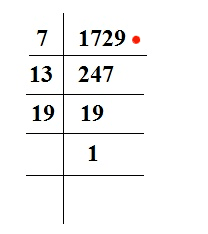These three (3) number are twin primes. We called the pairs as True Prime Pairs. Our scenario is mapping the distribution out of these pairs by taking the symmetrical behaviour of 36 as the smallest power (greater than 1) which is not a prime power.

Tip

The smallest square number expressible as the sum of four (4) consecutive primes in two ways (5 + 7 + 11 + 13 and 17 + 19) which are also two (2) couples of prime twins! (Prime Curios!).

``````\$True Prime Pairs:
(5,7), (11,13), (17,19)

layer|  i  |   f
-----+-----+---------
|  1  | 5
1  +-----+
|  2  | 7
-----+-----+---  } 36 » 6®
|  3  | 11
2  +-----+
|  4  | 13
-----+-----+---------
|  5  | 17
3  +-----+     } 36 » 6®
|  6  | 19
-----+-----+---------
``````

Thus in short this is all about the method that we called as the 19 vs 18 Scenario of mapping the quantum way within a huge of primes objects (5 to 19) by lexering (11) the ungrammared feed (7) and parsering (13) across syntax (17).

Φ(1,2,3) = Φ(6,12,18) = Φ(13,37,61)

``````\$True Prime Pairs:
(5,7), (11,13), (17,19)

layer | node | sub |  i  |  f
------+------+-----+----------
|      |     |  1  |
|      |  1  +-----+
|  1   |     |  2  | (5)
|      |-----+-----+
|      |     |  3  |
1   +------+  2  +-----+----
|      |     |  4  |
|      +-----+-----+
|  2   |     |  5  | (7)
|      |  3  +-----+
|      |     |  6  |
------+------+-----+-----+------      } (36)
|      |     |  7  |
|      |  4  +-----+
|  3   |     |  8  | (11)
|      +-----+-----+
|      |     |  9  |
2   +------|  5  +-----+-----
|      |     |  10 |
|      |-----+-----+
|  4   |     |  11 | (13)
|      |  6  +-----+
|      |     |  12 |
------+------+-----+-----+------------------
|      |     |  13 |
|      |  7  +-----+
|  5   |     |  14 | (17)
|      |-----+-----+
|      |     |  15 |
3   +------+  8  +-----+-----       } (36)
|      |     |  16 |
|      |-----+-----+
|  6   |     |  17 | (19)
|      |  9  +-----+
|      |     |  18 |
------|------|-----+-----+------
``````

The main background is that, as you may also aware, the prime number theorem describes the asymptotic distribution of prime numbers which is still a major problem in mathematic.

## Zeta Function

Instead of getting a proved formula we came to a unique expression called zeta function. This expression first appeared in a paper in 1737 entitled Variae observationes circa series infinitas.

Tip

This expression states that the sum of the zeta function is equal to the product of the reciprocal of one minus the reciprocal of primes to the power s. But what has this got to do with the primes? The answer is in the following product taken over the primes p (discovered by Leonhard Euler):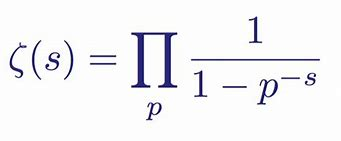This issue is actually come from Riemann hypothesis, a conjecture about the distribution of complex zeros of the Riemann zeta function that is considered by many mathematicians to be the most important of unsolved problems in pure mathematics.

Note

In addition to the trivial roots, there also exist complex roots for real t. We find that the he first ten (10) non-trivial roots of the Riemann zeta function is occured when the values of t below 50. A plot of the values of ζ(1/2 + it) for t ranging from –50 to +50 is shown below. The roots occur each time the locus passes through the origin. (mathpages).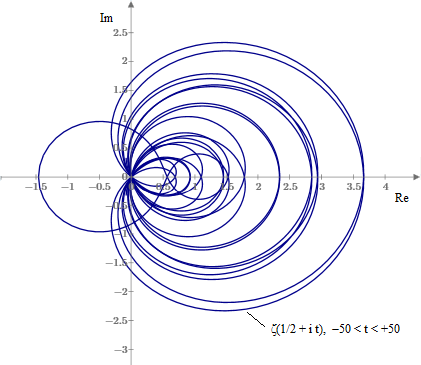Meanwhile obtaining the non complex numbers it is easier to look at a graph like the one below which shows Li(x) (blue), R(x) (black), π(x) (red) and x/ln x (green); and then proclaim "R(x) is the best estimate of π(x)." Indeed it is for that range, but as we mentioned above, Li(x)-π(x) changes sign infinitely often, and near where it does, Li(x) would be the best value.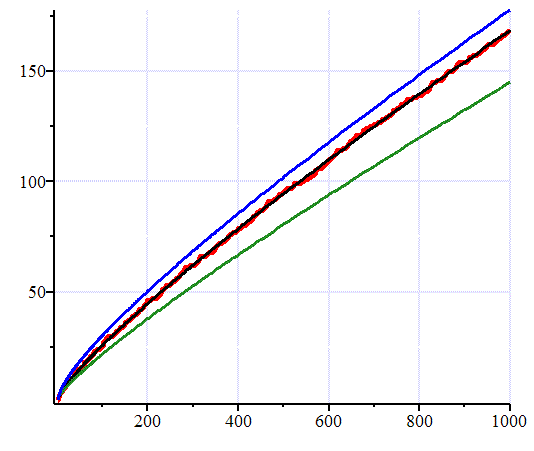And we can see in the same way that the function Li(x)-(1/2)Li(x1/2) is ‘on the average' a better approximation than Li(x) to π(x); but no importance can be attached to the latter terms in Riemann's formula even by repeated averaging. The problem is that the contributions from the non-trivial zeros at times swamps that of any but the main terms in these expansions.

Warning

A. E. Ingham says it this way: Considerable importance was attached formerly to a function suggested by Riemann as an approximation to π(x)… This function represents π(x) with astonishing accuracy for all values of x for which π(x) has been calculated, but we now see that its superiority over Li(x) is illusory… and for special values of x (as large as we please) the one approximation will deviate as widely as the other from the true value (primes.utm.edu).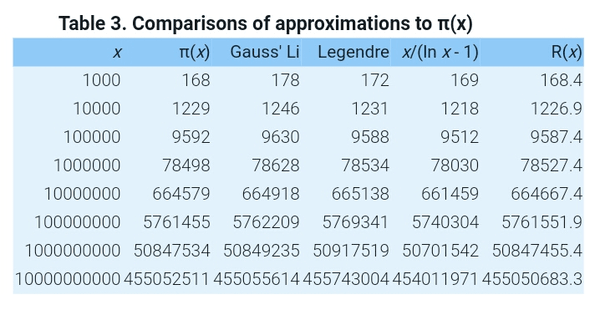Moreover in it was verified numerically, in a rigorous way using interval arithmetic, that The Riemann hypothesis is true up to 3 · 10^12. That is, all zeroes β+iγ of the Riemann zeta-function with 0<γ≤3⋅1012 have β=1/2.

Danger

We have Λ ≤ 0.2. The next entry is conditional on taking H a little higher than 10*13, which of course, is not achieved by Theorem 1. This would enable one to prove Λ < 0.19. Given that our value of H falls between the entries in this table, it is possible that some extra decimals could be wrought out of the calculation. We have not pursued this (arXiv:2004.09765).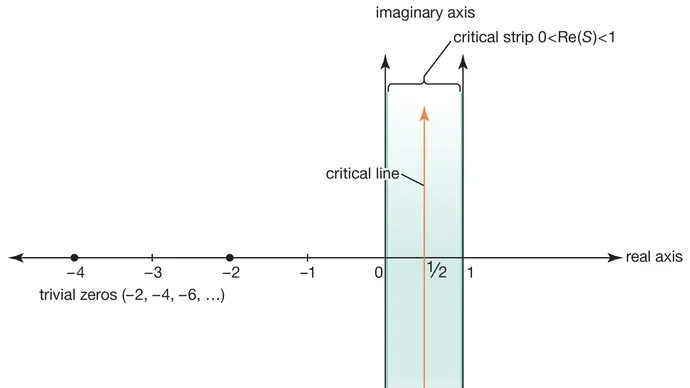This Euler formula represents the distribution of a group of numbers that are positioned at regular intervals on a straight line to each other. Riemann later extended the definition of zeta(s) to all complex numbers (except the simple pole at s=1 with residue one). Euler's product still holds if the real part of s is greater than one. Riemann derived the functional equation of zeta function.

Danger

The Riemann zeta function has the trivial zeros at -2, -4, -6, … (the poles of gamma(s/2)). Using the Euler product (with the functional equation) it is easy to show that all the other zeros are in the critical strip of non-real complex numbers with 0 < Re(s) < 1, and that they are symmetric about the critical line Re(s)=1/2. The unproved Riemann hypothesis is that all of the nontrivial zeros are actually on the critical line (primes.utm.edu).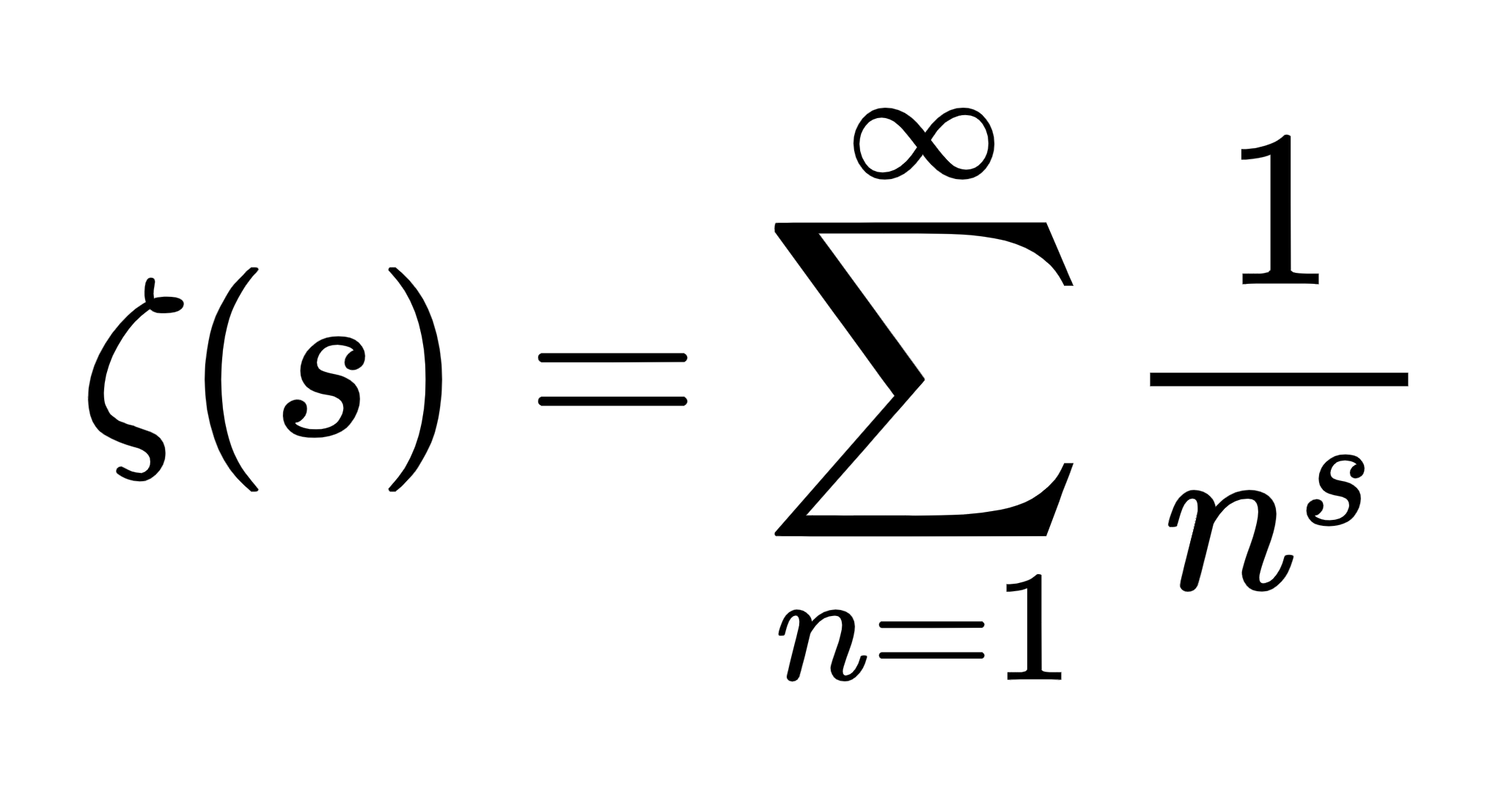If both of the above statements are true then mathematically this Riemann Hypothesis is proven to be incorrect because it only applies to certain cases or limitations. So first of all the basis of the Riemann Hypothesis has to be considered.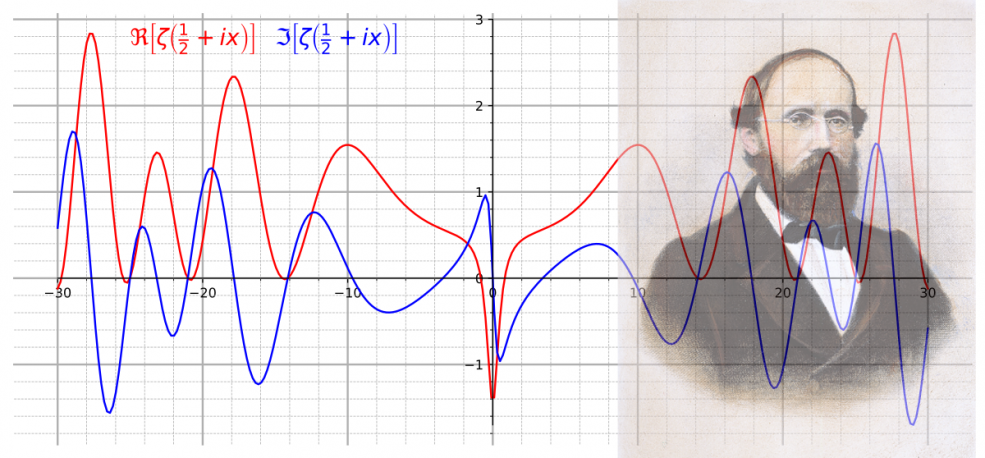Sehr leider Herr Riemann. Leute können den Fall immer noch nicht lösen...

On the other hand, the possibility of obtaining the function of the distribution of prime numbers shall go backwards since it needs significant studies to be traced. Or may be start again from the Euleur Function.

## Euler's identity

Freeman Dyson discovered an intriguing connection between quantum physics and Montgomery's pair correlation conjecture about the zeros of the zeta function which dealts with the distribution of primes.

Note

This scale shows that the Mathematical Elementary Cell 30 (MEC30) standard unites the mathematical and physical results of 1972 by the mathematician Hugh Montgomery and the physicist Freeman Dyson and thus reproduces energy distribution in systems as a path plan more accurately than a measurement (Google Patent DE102011101032A9).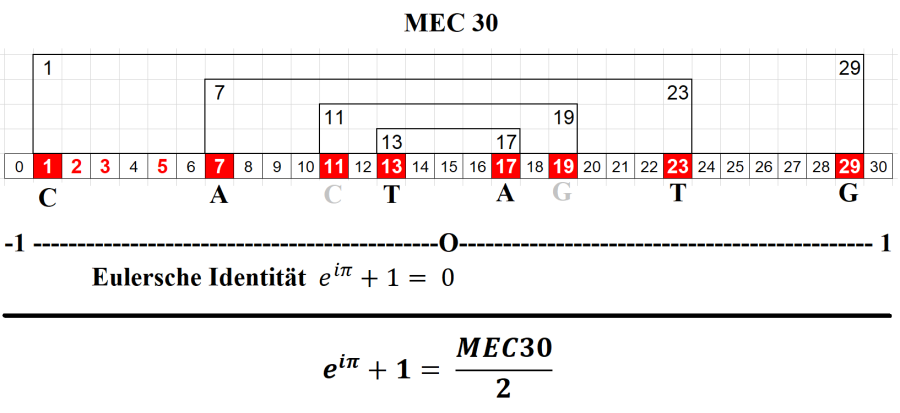Both assumptions assume a symmetric distribution of prime numbers with equal axial lengths from a middle zero axis = 15. With the MEC30 one is now able to determine the distribution of primes in a given number space. This finaly bring us to the equation of Euler's identity.

Note

Euler’s identity is named after the Swiss mathematician Leonhard Euler. It is a special case of Euler’s formula e^ix = cos x + i sin x when evaluated for x = π. (Wikipedia).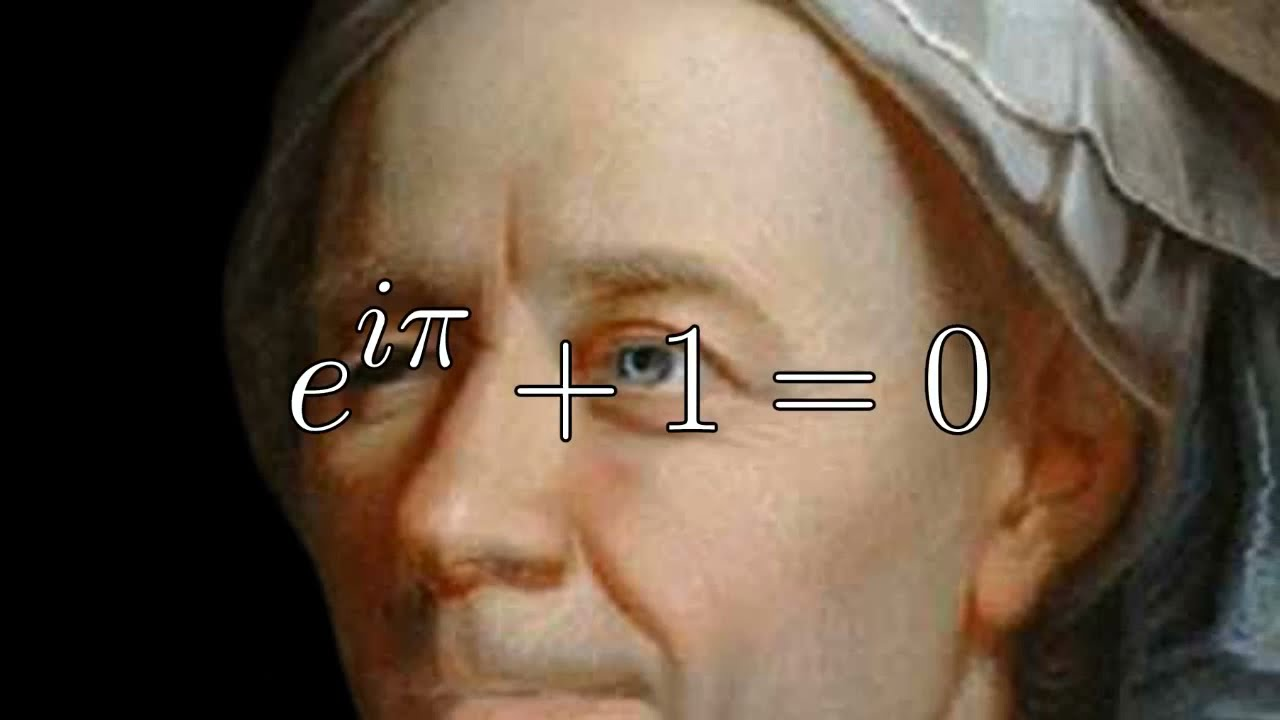The finiteness position of middle zero axis = 15 by the said MEC30 opens up the possibility of accurately representing the self-similarity based on the distribution of True Prime Pairs so that all number would belongs together with their own identitities.

Tip

Euler’s identity is considered to be an exemplar of deep mathematical beauty as it shows a profound connection between the most fundamental numbers. Three (3) of the basic arithmetic operations occur exactly once each: addition, multiplication, and exponentiation (Wikipedia).

Nothing is going to be easy about the nature of prime numbers but they demonstrably congruent to something organized. Let's discuss starting with the addition zones.